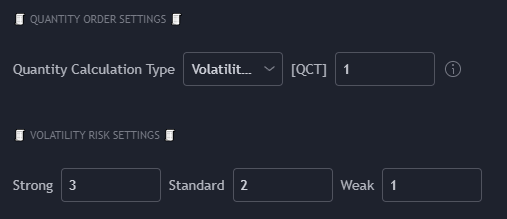Quantity Calculation Type

• Risk Based (default)
• Uses the Stop Loss value, Risk Percent Equity value, and current equity to calculate the quantity for a trade. This is a variable amount on each trade. Your position size is calculated using this formula:

Position Size = Equity * Risk % / Stop Loss %

• Fixed Percent
• Uses a static percent of your total equity to calculate the quantity to enter a trade.

• Fixed Dollar Amount
• Uses a fixed dollar value amount to calculate quantity to enter a trade.

• Volatility Based
• Uses fixed values to adjust your risk at 3 different "strength" volatility values using the Keltner Channel Bands indicator by ceyhun. Uses the same formula as "Risk Based" above to calculate your position sizes.
• The outer band is considered "strong", the one below is "standard", and the center is "weak". These terminologies apply to the strength of the Keltner Channels, not necessarily how much risk works best at these values. You may find lower risk at Strong and higher risk at Weak works better.